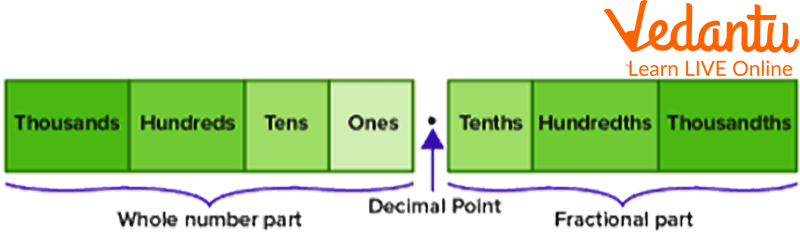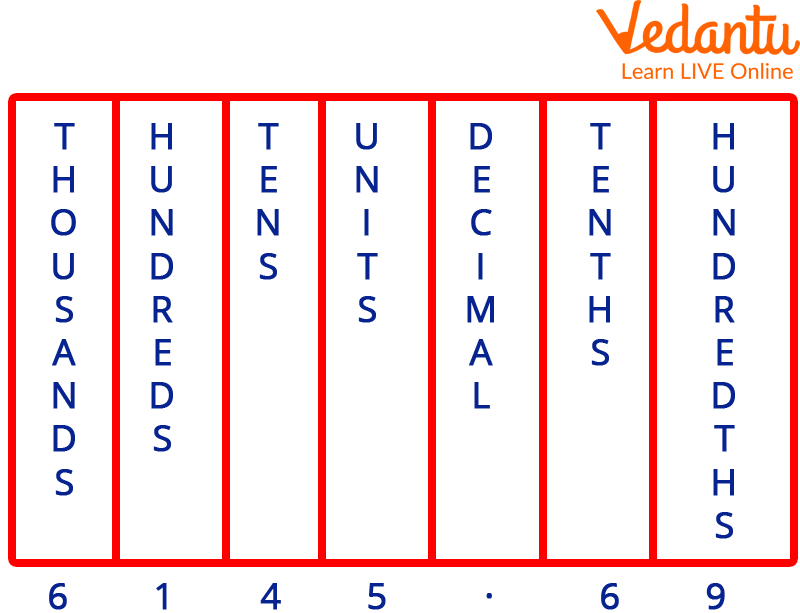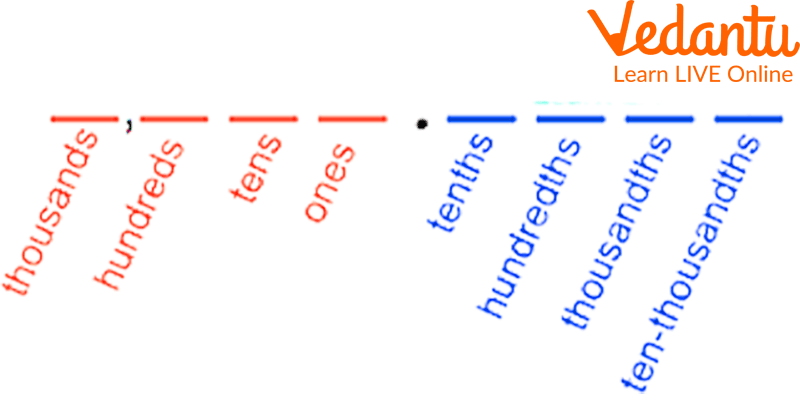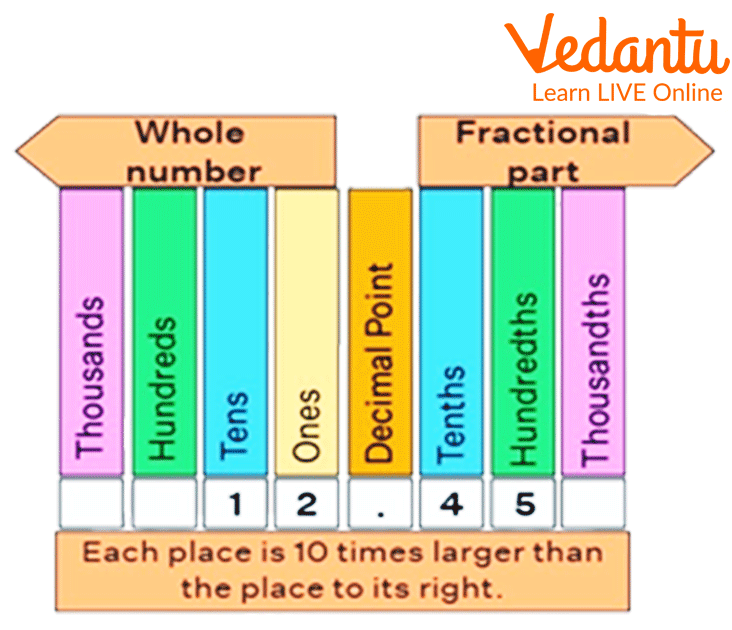Courses
Courses for Kids
Free study material
Free LIVE classes
More

# Decimal Place ValueLIVE
Join Vedantu’s FREE Mastercalss

## Introduction to Place Value in Decimal

The place values of the digits in a decimal number are displayed on the decimal place value chart. We know that a digit in a number represents a numerical value or place value.

Decimal place value charts are used to determine the proper placement of each digit in a decimal number. The place values of the digits given before and after the decimal point are displayed.Place Value in Decimal

## Place Value Definition in Decimals

A decimal number consists of a whole number and a fractional component, separated by the decimal point, a dot.

For instance, the decimal number 4.37 has two parts: an actual number portion of 4 and a fractional portion of 37. The place values of all digits are displayed in a decimal place value chart.This is How we Deduct Values after Decimal Points.

The place values often represented by the digits preceding the decimal point are ones, tens, hundreds, thousands, and so forth. While the place values represented by the numbers after the decimal point begin with the tenth, moving up through the hundredths and thousandth.Place Value Chart

## Chart of Decimal Place ValuesRepresentation of Decimal Values

The place values before the decimal point start with ones, followed by tens, and so forth, while the place values following the decimal point start from tenths, followed by hundredths, then thousandths, and so forth. The fractional portion of the number is represented by the place value that follows the decimal. The number 0.56, for instance, is composed of 5 tenths and 6 hundredths. This can be expressed as 0.56 = 0.5 + 0.06. In other terms, 0.56 is $\dfrac{5}{10} \text { plus } \dfrac{6}{100}$.

## Solved Examples

1. Write the place value of the digits 2 and 4 in the number 326.471

Ans: The steps to solve this problem is as follows:

• First, write the number in a decimal place value chart.

• Then, look at the digit's place and find its place value.

• The digit 2 is in the tens place. Therefore, its place value is 2 tens or 20.

• The digit 4 is in tenth place. Therefore, its place value is four-tenths of 0.4

2. Find the place value of the underlined digits in the number 4532.079

Ans: The steps to solve this problem is as follows:

• In the number 4532.079:

• 4 is at the thousands place. So, its place value is 4 thousands or 4000

• 0 is in tenth place. So, its place value is 0 tenths or 0

• 9 is at the thousandth place. So, its place value is 9 thousandths of 0.009

## Fun Facts About Decimal Place Value

• The actual value of a digit is called its face value. Unlike the place value of an integer, which depends upon its position in a number, the face value remains the same, irrespective of its class.

• Have you ever been told to multiply by ten, and add zero at the end? If so, you've been misled! Here's an example: 2.3 x 10 is not 2.30. It is 23.0. When you multiply by ten, every digit shifts along with one place to the left. So, in our example, there are 2 tens rather than 2.

• The 'dec' in decimal means ten and refers to the fact that each position in a decimal number corresponds to ten times more than the next. For example, the number 325.31 means 3 hundred, 2 tens, 5 ones, 3 tenths, and 1 hundredth. Humans decided to group in tens because that's how many fingers/thumbs we have. It made counting and arithmetic a whole lot easier. Three-fingered aliens might well group in threes!

## Practice Questions

Q 1. Write the place value of the digits 6 and 4 in the number 926.894

Ans: 6 = ones and 4 = thousandths

Q 2. Write the place value of the digit 2 in the number 73.42

Ans: Hundredths

## Summary

By adding decimal places, we can conclude that the base-10 number system may now express fractional numbers and unlimited amounts. To count parts or fractions of things, we utilize decimals. These are the numbers that appear on the number line in between the whole numbers. The decimal places are endlessly on the left and get smaller and smaller as you move along.

Last updated date: 30th Sep 2023
Total views: 126.3k
Views today: 1.26k

## FAQs on Decimal Place Value

1. Define the term "place value."

In mathematics, place value refers to a digit's location within a number. A number has a place for each digit. The placement of each digit will be expanded when we represent the number in a general form. These positions begin at the unit place, also known as the individual's position. Units, tens, hundreds, thousands, ten thousand, one hundred thousand, and so on are the place values of a number's digits from right to left.

2. What do you understand by a decimal?

The accepted method for representing both integer and non-integer numbers is the decimal numeral system. Decimal notation is the term used to describe the method of representing numbers in the decimal system. One sort of number that has a whole number and a fractional component separated by a decimal point is a decimal. The decimal point is the dot that appears between the parts of a whole number and a fraction. An example of a decimal number is 34.5.

3. What is the difference between place value and face value?

The value of each digit in a number is what is referred to as place value. By dividing the digit value by the numerical value of a number, we can determine its place value. Example: Since 3 is in the tens place, we multiply 3 (numerical value) by 10 (digit value) to get the place value of "3" in the number 45634. This gives us 30. The place values of the remaining digits in the number can also be determined in a similar manner. A number's digit's face value is that particular digit. For instance, the face value of "3" in the number 45634 is the number 3 itself.